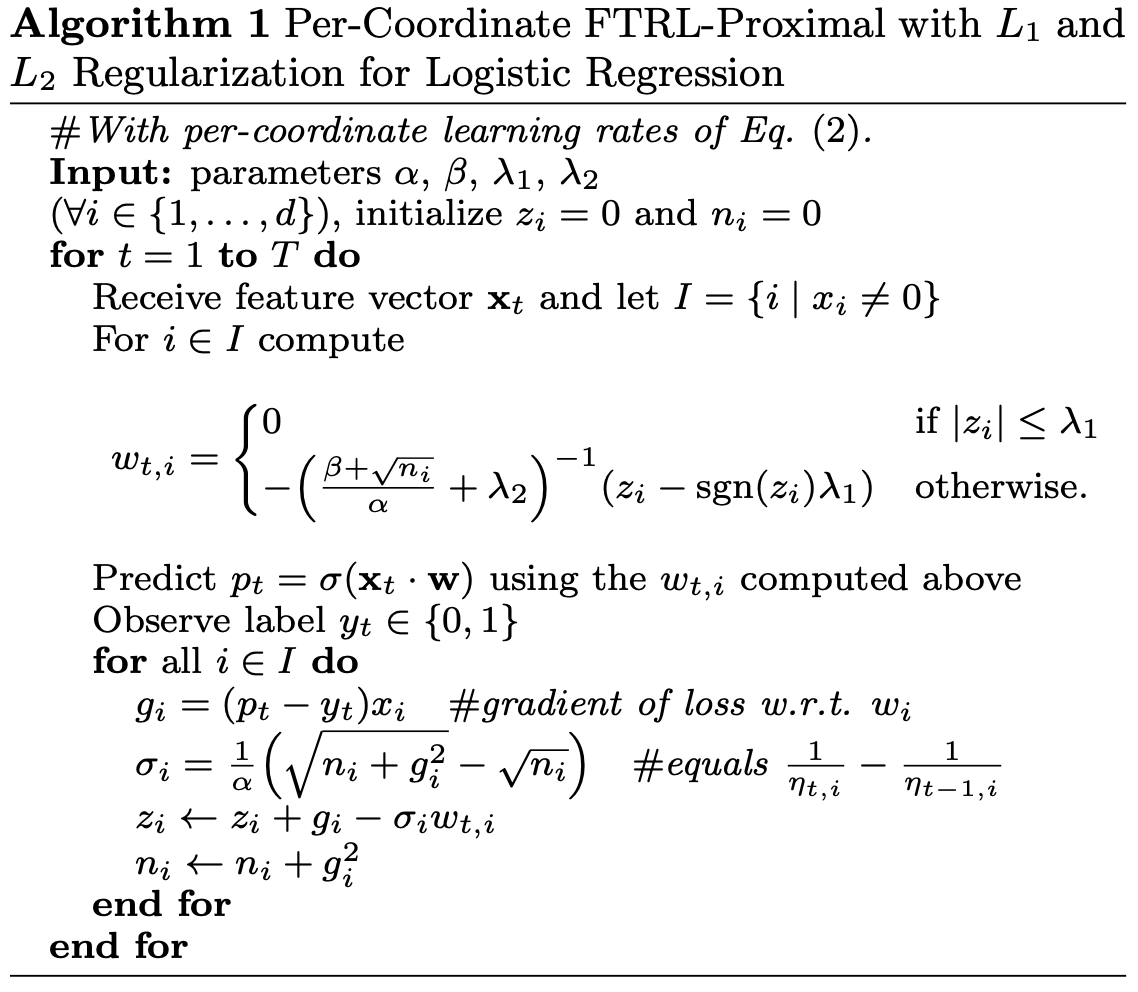# datatable.models.Ftrl¶

This class implements the Follow the Regularized Leader (FTRL) model, that is based on the FTRL-Proximal online learning algorithm for binomial logistic regression. Multinomial classification and regression for continuous targets are also implemented, though these implementations are experimental. This model is fully parallel and is based on the Hogwild approach for parallelization.

The model supports numerical (boolean, integer and float types), temporal (date and time types) and string features. To vectorize features a hashing trick is employed, such that all the values are hashed with the 64-bit hashing function. This function is implemented as follows:

• for booleans and integers the hashing function is essentially an identity function;

• for floats the hashing function trims mantissa, taking into account mantissa_nbits, and interprets the resulting bit representation as a 64-bit unsigned integer;

• for date and time types the hashing function is essentially an identity function that is based on their internal integer representations;

• for strings the 64-bit Murmur2 hashing function is used.

To compute the final hash x the Murmur2 hashed feature name is added to the hashed feature and the result is modulo divided by the number of requested bins, i.e. by nbins.

For each hashed row of data, according to Ad Click Prediction: a View from the Trenches, the following FTRL-Proximal algorithm is employed:When trained, the model can be used to make predictions, or it can be re-trained on new datasets as many times as needed improving model weights from run to run.

## Construction¶

 Ftrl() Construct an Ftrl object.

## Methods¶

 fit() Train model on the input samples and targets. predict() Predict for the input samples. reset() Reset the model.

## Properties¶

 alpha $$\alpha$$ in per-coordinate FTRL-Proximal algorithm. beta $$\beta$$ in per-coordinate FTRL-Proximal algorithm. colnames Column names of the training frame, i.e. features. colname_hashes Hashes of the column names. double_precision An option to control precision of the internal computations. feature_importances Feature importances calculated during training. interactions Feature interactions. labels Classification labels. lambda1 L1 regularization parameter, $$\lambda_1$$ in per-coordinate FTRL-Proximal algorithm. lambda2 L2 regularization parameter, $$\lambda_2$$ in per-coordinate FTRL-Proximal algorithm. mantissa_nbits Number of mantissa bits for hashing floats. model The model’s z and n coefficients. model_type A model type Ftrl should build. model_type_trained A model type Ftrl has built. nbins Number of bins for the hashing trick. negative_class An option to indicate if the “negative” class should be a created for multinomial classification. nepochs Number of training epochs. params All the input model parameters as a named tuple.